# Lorene::Tensor Class Reference [Tensorial fields]

Tensor handling. More...

#include <tensor.h>

Inheritance diagram for Lorene::Tensor: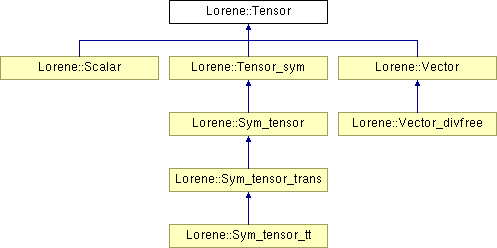List of all members.

## Public Member Functions

Tensor (const Map &map, int val, const Itbl &tipe, const Base_vect &triad_i)
Standard constructor.
Tensor (const Map &map, int val, const Itbl &tipe, const Base_vect *triad_i)
Standard constructor with the triad passed as a pointer.
Tensor (const Map &map, int val, int tipe, const Base_vect &triad_i)
Standard constructor when all the indices are of the same type.
Tensor (const Tensor &)
Copy constructor.
Tensor (const Map &map, const Base_vect &triad_i, FILE *fich)
Constructor from a file (see sauve(FILE*) ).
virtual ~Tensor ()
Destructor.
virtual void set_etat_nondef ()
Sets the logical state of all components to ETATNONDEF (undefined state).
virtual void set_etat_zero ()
Sets the logical state of all components to ETATZERO (zero state).
virtual void set_etat_qcq ()
Sets the logical state of all components to ETATQCQ (ordinary state).
virtual void allocate_all ()
Performs the memory allocation of all the elements, down to the double arrays of the Tbl s.
Sets a new vectorial basis (triad) of decomposition and modifies the components accordingly.
Assigns a new vectorial basis (triad) of decomposition.
virtual void operator= (const Tensor &)
Assignment to a Tensor.
Scalarset (const Itbl &ind)
Returns the value of a component (read/write version).
Scalarset (int i1, int i2)
Returns the value of a component for a tensor of valence 2 (read/write version).
Scalarset (int i1, int i2, int i3)
Returns the value of a component for a tensor of valence 3 (read/write version).
Scalarset (int i1, int i2, int i3, int i4)
Returns the value of a component for a tensor of valence 4 (read/write version).
void annule_domain (int l)
Sets the Tensor to zero in a given domain.
virtual void annule (int l_min, int l_max)
Sets the Tensor to zero in several domains.
void annule_extern_cn (int l_0, int deg)
Performs a smooth (C^n) transition in a given domain to zero.
virtual void std_spectral_base ()
Sets the standard spectal bases of decomposition for each component.
virtual void std_spectral_base_odd ()
Sets the standard odd spectal bases of decomposition for each component.
virtual void dec_dzpuis (int dec=1)
Decreases by dec units the value of dzpuis and changes accordingly the values in the compactified external domain (CED).
virtual void inc_dzpuis (int inc=1)
Increases by inc units the value of dzpuis and changes accordingly the values in the compactified external domain (CED).
virtual void exponential_filter_r (int lzmin, int lzmax, int p, double alpha=-16.)
Applies exponential filters to all components (see Scalar::exponential_filter_r ).
virtual void exponential_filter_ylm (int lzmin, int lzmax, int p, double alpha=-16.)
Applies exponential filters to all components (see Scalar::exponential_filter_ylm ).
const Tensorderive_cov (const Metric &gam) const
Returns the covariant derivative of this with respect to some metric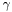.
const Tensorderive_con (const Metric &gam) const
Returns the "contravariant" derivative of this with respect to some metric, by raising the last index of the covariant derivative (cf.
const Tensordivergence (const Metric &gam) const
Computes the divergence of this with respect to some metric.
Tensor derive_lie (const Vector &v) const
Computes the Lie derivative of this with respect to some vector field v.
Tensor up (int ind, const Metric &gam) const
Computes a new tensor by raising an index of *this.
Tensor down (int ind, const Metric &gam) const
Computes a new tensor by lowering an index of *this.
Tensor up_down (const Metric &gam) const
Computes a new tensor by raising or lowering all the indices of *this .
Tensor trace (int ind1, int ind2) const
Trace on two different type indices.
Tensor trace (int ind1, int ind2, const Metric &gam) const
Trace with respect to a given metric.
Scalar trace () const
Trace on two different type indices for a valence 2 tensor.
Scalar trace (const Metric &gam) const
Trace with respect to a given metric for a valence 2 tensor.
virtual int position (const Itbl &ind) const
Returns the position in the array cmp of a component given by its indices.
virtual Itbl indices (int pos) const
Returns the indices of a component given by its position in the array cmp .
const Mapget_mp () const
Returns the mapping.
Returns the vectorial basis (triad) on which the components are defined.
int get_valence () const
Returns the valence.
int get_n_comp () const
Returns the number of stored components.
int get_index_type (int i) const
Gives the type (covariant or contravariant) of the index number i .
Itbl get_index_type () const
Returns the types of all the indices.
int & set_index_type (int i)
Sets the type of the index number i .
Itblset_index_type ()
Sets the types of all the indices.
const Scalaroperator() (const Itbl &ind) const
Returns the value of a component (read-only version).
const Scalaroperator() (int i1, int i2) const
Returns the value of a component for a tensor of valence 2 (read-only version).
const Scalaroperator() (int i1, int i2, int i3) const
Returns the value of a component for a tensor of valence 3 (read-only version).
const Scalaroperator() (int i1, int i2, int i3, int i4) const
Returns the value of a component for a tensor of valence 4 (read-only version).
void operator+= (const Tensor &)
+= Tensor
void operator-= (const Tensor &)
-= Tensor
virtual void sauve (FILE *) const
Save in a binary file.
virtual void spectral_display (const char *comment=0x0, double threshold=1.e-7, int precision=4, ostream &ostr=cout) const
Displays the spectral coefficients and the associated basis functions of each component.

## Protected Member Functions

Tensor (const Map &map)
Constructor for a scalar field: to be used only by the derived class Scalar .
Tensor (const Map &map, int val, const Itbl &tipe, int n_comp_i, const Base_vect &triad_i)
Constructor to be used by derived classes, with symmetries among the components.
Tensor (const Map &map, int val, int tipe, int n_comp_i, const Base_vect &triad_i)
Constructor used by derived classes, with symmetries among the components, when all the indices are of the same type.
virtual void del_deriv () const
Deletes the derived quantities.
void set_der_0x0 () const
Sets the pointers on derived quantities to 0x0.
virtual void del_derive_met (int) const
Logical destructor of the derivatives depending on the i-th element of met_depend .
void set_der_met_0x0 (int) const
Sets all the i-th components of met_depend , p_derive_cov , etc.
void set_dependance (const Metric &) const
To be used to describe the fact that the derivatives members have been calculated with met .
int get_place_met (const Metric &) const
Returns the position of the pointer on metre in the array met_depend .
void compute_derive_lie (const Vector &v, Tensor &resu) const
Computes the Lie derivative of this with respect to some vector field v (protected method; the public interface is method derive_lie ).

## Protected Attributes

const Map *const mp
Mapping on which the numerical values at the grid points are defined.
int valence
Valence of the tensor (0 = scalar, 1 = vector, etc...).
Vectorial basis (triad) with respect to which the tensor components are defined.
Itbl type_indice
1D array of integers (class Itbl ) of size valence containing the type of each index: COV for a covariant one and CON for a contravariant one.
int n_comp
Number of stored components, depending on the symmetry.
Scalar ** cmp
Array of size n_comp of pointers onto the components.
const Metricmet_depend [N_MET_MAX]
Array on the Metric 's which were used to compute derived quantities, like p_derive_cov , etc.
Tensorp_derive_cov [N_MET_MAX]
Array of pointers on the covariant derivatives of this with respect to various metrics.
Tensorp_derive_con [N_MET_MAX]
Array of pointers on the contravariant derivatives of this with respect to various metrics.
Tensorp_divergence [N_MET_MAX]
Array of pointers on the divergence of this with respect to various metrics.

## Friends

class Scalar
class Vector
class Sym_tensor
class Tensor_sym
class Metric
ostream & operator<< (ostream &, const Tensor &)
Scalar operator+ (const Tensor &, const Scalar &)
Scalar operator+ (const Scalar &, const Tensor &)
Scalar operator- (const Tensor &, const Scalar &)
Scalar operator- (const Scalar &, const Tensor &)
Tensor operator* (const Tensor &, const Tensor &)
Tensor_sym operator* (const Tensor &, const Tensor_sym &)
Tensor_sym operator* (const Tensor_sym &, const Tensor &)
Tensor_sym operator* (const Tensor_sym &, const Tensor_sym &)
Tensorial product of two symmetric tensors.

## Detailed Description

Tensor handling.

()

This class has been devised to replace Tenseur and Cmp (the latter via the derived class Scalar ).

The Tensor class is intended to store the components of a tensorial field with respect to a specific basis (triad).

All this is 3D meaning that the indices go from 1 to 3.

Definition at line 288 of file tensor.h.

## Constructor & Destructor Documentation

 Lorene::Tensor::Tensor ( const Map & map, int val, const Itbl & tipe, const Base_vect & triad_i )

Standard constructor.

Parameters:
 map the mapping val valence of the tensor tipe 1-D array of integers (class Itbl ) of size valence containing the type of each index, COV for a covariant one and CON for a contravariant one, with the following storage convention: tipe(0) : type of the first index tipe(1) : type of the second index and so on... triad_i vectorial basis (triad) with respect to which the tensor components are defined

Definition at line 205 of file tensor.C.

References cmp, Lorene::Itbl::get_dim(), Lorene::Itbl::get_ndim(), n_comp, set_der_0x0(), and valence.

 Lorene::Tensor::Tensor ( const Map & map, int val, const Itbl & tipe, const Base_vect * triad_i )

Standard constructor with the triad passed as a pointer.

Parameters:
 map the mapping val valence of the tensor tipe 1-D array of integers (class Itbl ) of size valence containing the type of each index, COV for a covariant one and CON for a contravariant one, with the following storage convention: tipe(0) : type of the first index tipe(1) : type of the second index and so on... triad_i pointer on the vectorial basis (triad) with respect to which the tensor components are defined (can be set to 0x0 for a scalar field)

Definition at line 228 of file tensor.C.

References cmp, Lorene::Itbl::get_dim(), Lorene::Itbl::get_ndim(), n_comp, set_der_0x0(), and valence.

 Lorene::Tensor::Tensor ( const Map & map, int val, int tipe, const Base_vect & triad_i )

Standard constructor when all the indices are of the same type.

Parameters:
 map the mapping val valence of the tensor tipe the type (COV or CON ) of the indices. triad_i vectorial basis (triad) with respect to which the tensor components are defined.

Definition at line 254 of file tensor.C.

References cmp, n_comp, set_der_0x0(), type_indice, and valence.

 Lorene::Tensor::Tensor ( const Tensor & source )

Copy constructor.

Definition at line 274 of file tensor.C.

References cmp, indices(), n_comp, position(), Lorene::pow(), set_der_0x0(), and valence.

 Lorene::Tensor::Tensor ( const Map & map, const Base_vect & triad_i, FILE * fich )

Constructor from a file (see sauve(FILE*) ).

Parameters:
 map the mapping triad_i vectorial basis (triad) with respect to which the tensor components are defined. It will be checked that it coincides with the basis saved in the file. fich file which has been created by the function sauve(FILE*) .

Definition at line 298 of file tensor.C.

 Lorene::Tensor::Tensor ( const Map & map )  [explicit, protected]

Constructor for a scalar field: to be used only by the derived class Scalar .

Definition at line 325 of file tensor.C.

References cmp, n_comp, and set_der_0x0().

 Lorene::Tensor::Tensor ( const Map & map, int val, const Itbl & tipe, int n_comp_i, const Base_vect & triad_i )  [protected]

Constructor to be used by derived classes, with symmetries among the components.

The number of independent components is given as an argument (n_comp_i ), and not computed from the valence, as in the standard constructor.

Parameters:
 map the mapping val valence of the tensor tipe 1-D array of integers (class Itbl ) of size valence containing the type of each index, COV for a covariant one and CON for a contravariant one, with the following storage convention: tipe(0) : type of the first index tipe(1) : type of the second index and so on... n_comp_i number of components to be stored triad_i vectorial basis (triad) with respect to which the tensor components are defined

Definition at line 337 of file tensor.C.

References cmp, Lorene::Itbl::get_dim(), Lorene::Itbl::get_ndim(), n_comp, set_der_0x0(), and valence.

 Lorene::Tensor::Tensor ( const Map & map, int val, int tipe, int n_comp_i, const Base_vect & triad_i )  [protected]

Constructor used by derived classes, with symmetries among the components, when all the indices are of the same type.

The number of independent components is given as a argument (n_comp_i ), and not computed from the valence, as in the standard constructor.

Parameters:
 map the mapping val valence of the tensor tipe the type of the indices. n_comp_i number of components to be stored triad_i vectorial basis (triad) with respect to which the tensor components are defined

Definition at line 362 of file tensor.C.

References cmp, n_comp, set_der_0x0(), type_indice, and valence.

 Lorene::Tensor::~Tensor ( )  [virtual]

Destructor.

Definition at line 388 of file tensor.C.

References cmp, del_deriv(), and n_comp.

## Member Function Documentation

 void Lorene::Tensor::allocate_all ( )  [virtual]

Performs the memory allocation of all the elements, down to the double arrays of the Tbl s.

This function performs in fact recursive calls to set_etat_qcq() on each element of the chain Scalar -> Valeur -> Mtbl -> Tbl .

Reimplemented in Lorene::Scalar.

Definition at line 511 of file tensor.C.

References Lorene::Scalar::allocate_all(), cmp, del_deriv(), and n_comp.

 void Lorene::Tensor::annule ( int l_min, int l_max )  [virtual]

Sets the Tensor to zero in several domains.

Parameters:
 l_min [input] The Tensor will be set (logically) to zero in the domains whose indices are in the range [l_min,l_max] . l_max [input] see the comments for l_min .

Note that annule(0,nz-1) , where nz is the total number of domains, is equivalent to set_etat_zero() .

Reimplemented in Lorene::Scalar.

Definition at line 674 of file tensor.C.

 void Lorene::Tensor::annule_domain ( int l )

Sets the Tensor to zero in a given domain.

Parameters:
 l [input] Index of the domain in which the Tensor will be set (logically) to zero.

Definition at line 669 of file tensor.C.

References annule().

 void Lorene::Tensor::annule_extern_cn ( int l_0, int deg )

Performs a smooth (C^n) transition in a given domain to zero.

Parameters:
 l_0 [input] in the domain of index l0 the tensor is multiplied by the right polynomial (of degree 2n+1), to ensure continuty of the function and its n first derivative at both ends of this domain. The tensor is unchanged in the domains l < l_0 and set to zero in domains l > l_0. deg [input] the degree n of smoothness of the transition.

Definition at line 693 of file tensor.C.

 void Lorene::Tensor::change_triad ( const Base_vect & new_triad )  [virtual]

Sets a new vectorial basis (triad) of decomposition and modifies the components accordingly.

Reimplemented in Lorene::Scalar, and Lorene::Vector.

Definition at line 80 of file tensor_change_triad.C.

 void Lorene::Tensor::compute_derive_lie ( const Vector & v, Tensor & resu ) const [protected]

Computes the Lie derivative of this with respect to some vector field v (protected method; the public interface is method derive_lie ).

Definition at line 342 of file tensor_calculus.C.

 void Lorene::Tensor::dec_dzpuis ( int dec = 1 )  [virtual]

Decreases by dec units the value of dzpuis and changes accordingly the values in the compactified external domain (CED).

Reimplemented in Lorene::Scalar.

Definition at line 811 of file tensor.C.

References cmp, del_deriv(), and n_comp.

 void Lorene::Tensor::del_deriv ( ) const [protected, virtual]

Deletes the derived quantities.

Definition at line 401 of file tensor.C.

References del_derive_met(), and set_der_0x0().

 void Lorene::Tensor::del_derive_met ( int j ) const [protected, virtual]

Logical destructor of the derivatives depending on the i-th element of met_depend .

Reimplemented in Lorene::Sym_tensor, and Lorene::Vector.

Definition at line 417 of file tensor.C.

 const Tensor & Lorene::Tensor::derive_con ( const Metric & gam ) const

Returns the "contravariant" derivative of this with respect to some metric, by raising the last index of the covariant derivative (cf.

method derive_cov() ) with.

Reimplemented in Lorene::Scalar, and Lorene::Tensor_sym.

Definition at line 1017 of file tensor.C.

 const Tensor & Lorene::Tensor::derive_cov ( const Metric & gam ) const

Returns the covariant derivative of this with respect to some metric.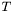denoting the tensor represented by this and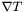its covariant derivative with respect to the metric, the extra index (with respect to the indices of) ofis chosen to be the last one. This convention agrees with that of MTW (see Eq. (10.17) of MTW). For instance, ifis a 1-form, whose components w.r.t. the triad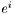are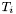: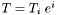, then the covariant derivative ofis the bilinear formwhose components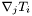are such that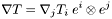Parameters:
 gam metricReturns:
covariant derivativeof this with respect to the connection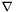associated with the metricReimplemented in Lorene::Scalar, and Lorene::Tensor_sym.

Definition at line 1005 of file tensor.C.

 Tensor Lorene::Tensor::derive_lie ( const Vector & v ) const

Computes the Lie derivative of this with respect to some vector field v.

Reimplemented in Lorene::Scalar, Lorene::Sym_tensor, and Lorene::Tensor_sym.

Definition at line 484 of file tensor_calculus.C.

References compute_derive_lie(), mp, triad, type_indice, and valence.

 const Tensor & Lorene::Tensor::divergence ( const Metric & gam ) const

Computes the divergence of this with respect to some metric.

The divergence is taken with respect of the last index of this which thus must be contravariant. For instance if the tensorrepresented by this is a twice contravariant tensor, whose components w.r.t. the triad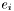are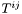: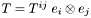, the divergence ofw.r.t.is the vector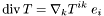wheredenotes the connection associated with the metric.

Parameters:
 gam metricReturns:
divergence of this with respect to.

Reimplemented in Lorene::Sym_tensor, and Lorene::Vector.

Definition at line 1058 of file tensor.C.

 Tensor Lorene::Tensor::down ( int ind, const Metric & gam ) const

Computes a new tensor by lowering an index of *this.

Parameters:
 ind index to be lowered, with the following convention : ind1 = 0 : first index of the tensor ind1 = 1 : second index of the tensor and so on... (ind must be of covariant type (CON )). gam metric used to lower the index (contraction with the twice covariant form of the metric on the index ind ).

Definition at line 268 of file tensor_calculus.C.

 void Lorene::Tensor::exponential_filter_r ( int lzmin, int lzmax, int p, double alpha = -16. )  [virtual]

Applies exponential filters to all components (see Scalar::exponential_filter_r ).

Works only for Cartesian components.

Reimplemented in Lorene::Scalar, Lorene::Sym_tensor, and Lorene::Vector.

Definition at line 1069 of file tensor.C.

References cmp, Lorene::Map::get_bvect_cart(), Lorene::Base_vect::identify(), mp, n_comp, and triad.

 void Lorene::Tensor::exponential_filter_ylm ( int lzmin, int lzmax, int p, double alpha = -16. )  [virtual]

Applies exponential filters to all components (see Scalar::exponential_filter_ylm ).

Works only for Cartesian components.

Reimplemented in Lorene::Scalar, Lorene::Sym_tensor, and Lorene::Vector.

Definition at line 1082 of file tensor.C.

References cmp, Lorene::Map::get_bvect_cart(), Lorene::Base_vect::identify(), mp, n_comp, and triad.

 Itbl Lorene::Tensor::get_index_type ( ) const [inline]

Returns the types of all the indices.

Returns:
1-D array of integers (class Itbl ) of size valence containing the type of each index, COV for a covariant one and CON for a contravariant one.

Definition at line 896 of file tensor.h.

References type_indice.

 int Lorene::Tensor::get_index_type ( int i ) const [inline]

Gives the type (covariant or contravariant) of the index number i .

i must be strictly lower than valence and obey the following convention:

• i = 0 : first index
• i = 1 : second index
• and so on...
Returns:
COV for a covariant index, CON for a contravariant one.

Definition at line 886 of file tensor.h.

References type_indice.

 const Map& Lorene::Tensor::get_mp ( ) const [inline]

Returns the mapping.

Definition at line 861 of file tensor.h.

References mp.

 int Lorene::Tensor::get_n_comp ( ) const [inline]

Returns the number of stored components.

Definition at line 872 of file tensor.h.

References n_comp.

 int Lorene::Tensor::get_place_met ( const Metric & metre ) const [protected]

Returns the position of the pointer on metre in the array met_depend .

Definition at line 446 of file tensor.C.

References met_depend.

 const Base_vect* Lorene::Tensor::get_triad ( ) const [inline]

Returns the vectorial basis (triad) on which the components are defined.

Definition at line 866 of file tensor.h.

 int Lorene::Tensor::get_valence ( ) const [inline]

Returns the valence.

Definition at line 869 of file tensor.h.

References valence.

 void Lorene::Tensor::inc_dzpuis ( int inc = 1 )  [virtual]

Increases by inc units the value of dzpuis and changes accordingly the values in the compactified external domain (CED).

Reimplemented in Lorene::Scalar.

Definition at line 819 of file tensor.C.

References cmp, del_deriv(), and n_comp.

 Itbl Lorene::Tensor::indices ( int pos ) const [virtual]

Returns the indices of a component given by its position in the array cmp .

Parameters:
 pos [input] position in the array cmp of the pointer to the Scalar representing a component
Returns:
1-D array of integers (class Itbl ) of size valence giving the value of each index for the component located at the position pos in the array cmp, with the following storage convention:
• Itbl(0) : value of the first index (1, 2 or 3)
• Itbl(1) : value of the second index (1, 2 or 3)
• and so on...

Reimplemented in Lorene::Tensor_sym, and Lorene::Vector.

Definition at line 542 of file tensor.C.

References n_comp, Lorene::Itbl::set(), and valence.

 const Scalar & Lorene::Tensor::operator() ( int i1, int i2, int i3, int i4 ) const

Returns the value of a component for a tensor of valence 4 (read-only version).

Parameters:
 i1 value of the first index (1, 2 or 3) i2 value of the second index (1, 2 or 3) i3 value of the third index (1, 2 or 3) i4 value of the fourth index (1, 2 or 3)
Returns:
reference on the component specified by (i1,i2,i3,i4)

Definition at line 786 of file tensor.C.

References cmp, position(), Lorene::Itbl::set(), and valence.

 const Scalar & Lorene::Tensor::operator() ( int i1, int i2, int i3 ) const

Returns the value of a component for a tensor of valence 3 (read-only version).

Parameters:
 i1 value of the first index (1, 2 or 3) i2 value of the second index (1, 2 or 3) i3 value of the third index (1, 2 or 3)
Returns:
reference on the component specified by (i1,i2,i3)

Definition at line 774 of file tensor.C.

References cmp, position(), Lorene::Itbl::set(), and valence.

 const Scalar & Lorene::Tensor::operator() ( int i1, int i2 ) const

Returns the value of a component for a tensor of valence 2 (read-only version).

Parameters:
 i1 value of the first index (1, 2 or 3) i2 value of the second index (1, 2 or 3)
Returns:
reference on the component specified by (i1,i2)

Definition at line 763 of file tensor.C.

References cmp, position(), Lorene::Itbl::set(), and valence.

 const Scalar & Lorene::Tensor::operator() ( const Itbl & ind ) const

Returns the value of a component (read-only version).

Parameters:
 ind 1-D Itbl of size valence containing the values of each index specifing the component, with the following storage convention: ind(0) : value of the first index (1, 2 or 3) ind(1) : value of the second index (1, 2 or 3) and so on...
Returns:
reference on the component specified by ind

Definition at line 801 of file tensor.C.

References cmp, Lorene::Itbl::get_dim(), Lorene::Itbl::get_ndim(), position(), and valence.

 void Lorene::Tensor::operator+= ( const Tensor & t )

+= Tensor

Reimplemented in Lorene::Scalar.

Definition at line 574 of file tensor.C.

References cmp, del_deriv(), indices(), n_comp, position(), triad, type_indice, and valence.

 void Lorene::Tensor::operator-= ( const Tensor & t )

-= Tensor

Reimplemented in Lorene::Scalar.

Definition at line 590 of file tensor.C.

References cmp, del_deriv(), indices(), n_comp, position(), triad, type_indice, and valence.

 void Lorene::Tensor::operator= ( const Tensor & t )  [virtual]

Assignment to a Tensor.

Definition at line 556 of file tensor.C.

References cmp, del_deriv(), indices(), n_comp, position(), triad, type_indice, and valence.

 int Lorene::Tensor::position ( const Itbl & ind ) const [virtual]

Returns the position in the array cmp of a component given by its indices.

Parameters:
 ind [input] 1-D array of integers (class Itbl ) of size valence giving the values of each index specifing the component, with the following storage convention: ind(0) : value of the first index (1, 2 or 3) ind(1) : value of the second index (1, 2 or 3) and so on...
Returns:
position in the array cmp of the pointer to the Scalar containing the component specified by ind

Reimplemented in Lorene::Tensor_sym, and Lorene::Vector.

Definition at line 528 of file tensor.C.

References Lorene::Itbl::get_dim(), Lorene::Itbl::get_ndim(), and valence.

 void Lorene::Tensor::sauve ( FILE * fd ) const [virtual]

Save in a binary file.

Reimplemented in Lorene::Scalar, and Lorene::Tensor_sym.

Definition at line 909 of file tensor.C.

 Scalar & Lorene::Tensor::set ( int i1, int i2, int i3, int i4 )

Returns the value of a component for a tensor of valence 4 (read/write version).

Parameters:
 i1 value of the first index (1, 2 or 3) i2 value of the second index (1, 2 or 3) i3 value of the third index (1, 2 or 3) i4 value of the fourth index (1, 2 or 3)
Returns:
modifiable reference on the component specified by (i1,i2,i3,i4)

Definition at line 640 of file tensor.C.

References cmp, del_deriv(), position(), Lorene::Itbl::set(), and valence.

 Scalar & Lorene::Tensor::set ( int i1, int i2, int i3 )

Returns the value of a component for a tensor of valence 3 (read/write version).

Parameters:
 i1 value of the first index (1, 2 or 3) i2 value of the second index (1, 2 or 3) i3 value of the third index (1, 2 or 3)
Returns:
modifiable reference on the component specified by (i1,i2,i3)

Definition at line 624 of file tensor.C.

References cmp, del_deriv(), position(), Lorene::Itbl::set(), and valence.

 Scalar & Lorene::Tensor::set ( int i1, int i2 )

Returns the value of a component for a tensor of valence 2 (read/write version).

Parameters:
 i1 value of the first index (1, 2 or 3) i2 value of the second index (1, 2 or 3)
Returns:
modifiable reference on the component specified by (i1,i2)

Definition at line 609 of file tensor.C.

References cmp, del_deriv(), position(), Lorene::Itbl::set(), and valence.

 Scalar & Lorene::Tensor::set ( const Itbl & ind )

Returns the value of a component (read/write version).

Parameters:
 ind 1-D Itbl of size valence containing the values of each index specifing the component, with the following storage convention: ind(0) : value of the first index (1, 2 or 3) ind(1) : value of the second index (1, 2 or 3) and so on...
Returns:
modifiable reference on the component specified by ind

Definition at line 657 of file tensor.C.

References cmp, del_deriv(), Lorene::Itbl::get_dim(), Lorene::Itbl::get_ndim(), position(), and valence.

 void Lorene::Tensor::set_dependance ( const Metric & met ) const [protected]

To be used to describe the fact that the derivatives members have been calculated with met .

First it sets a null element of met_depend to &met and puts this in the list of the dependancies of met .

Definition at line 456 of file tensor.C.

References met_depend, and Lorene::Metric::tensor_depend.

 void Lorene::Tensor::set_der_0x0 ( ) const [protected]

Sets the pointers on derived quantities to 0x0.

Definition at line 410 of file tensor.C.

References set_der_met_0x0().

 void Lorene::Tensor::set_der_met_0x0 ( int i ) const [protected]

Sets all the i-th components of met_depend , p_derive_cov , etc.

.. to 0x0.

Reimplemented in Lorene::Sym_tensor, and Lorene::Vector.

Definition at line 436 of file tensor.C.

References met_depend, p_derive_con, p_derive_cov, and p_divergence.

 void Lorene::Tensor::set_etat_nondef ( )  [virtual]

Sets the logical state of all components to ETATNONDEF (undefined state).

Reimplemented in Lorene::Scalar.

Definition at line 492 of file tensor.C.

References cmp, del_deriv(), n_comp, and Lorene::Scalar::set_etat_nondef().

 void Lorene::Tensor::set_etat_qcq ( )  [virtual]

Sets the logical state of all components to ETATQCQ (ordinary state).

Reimplemented in Lorene::Scalar.

Definition at line 484 of file tensor.C.

References cmp, del_deriv(), n_comp, and Lorene::Scalar::set_etat_qcq().

 void Lorene::Tensor::set_etat_zero ( )  [virtual]

Sets the logical state of all components to ETATZERO (zero state).

Reimplemented in Lorene::Scalar.

Definition at line 500 of file tensor.C.

References cmp, del_deriv(), n_comp, and Lorene::Scalar::set_etat_zero().

 Itbl& Lorene::Tensor::set_index_type ( )  [inline]

Sets the types of all the indices.

Returns:
a reference on the 1-D array of integers (class Itbl ) of size valence that can be modified (COV for a covariant one and CON for a contravariant one)

Definition at line 918 of file tensor.h.

References type_indice.

 int& Lorene::Tensor::set_index_type ( int i )  [inline]

Sets the type of the index number i .

i must be strictly lower than valence and obey the following convention:

• i = 0 : first index
• i = 1 : second index
• and so on...
Returns:
reference on the type that can be modified (COV for a covariant index, CON for a contravariant one)

Definition at line 909 of file tensor.h.

References Lorene::Itbl::set(), and type_indice.

Assigns a new vectorial basis (triad) of decomposition.

NB: this function modifies only the member triad and leave unchanged the components (member cmp ). In order to change them coherently with the new basis, the function change_triad(const Base_vect& ) must be called instead.

Definition at line 522 of file tensor.C.

 void Lorene::Tensor::spectral_display ( const char * comment = 0x0, double threshold = 1.e-7, int precision = 4, ostream & ostr = cout ) const [virtual]

Displays the spectral coefficients and the associated basis functions of each component.

This function shows only the values greater than a given threshold.

Parameters:
 comment comment to be printed at top of the display (default: 0x0 = nothing printed) threshold [input] Value above which a coefficient is printed (default: 1.e-7) precision [input] Number of printed digits (default: 4) ostr [input] Output stream used for the printing (default: cout)

Reimplemented in Lorene::Scalar.

Definition at line 877 of file tensor.C.

References cmp, indices(), n_comp, Lorene::Scalar::spectral_display(), and valence.

 void Lorene::Tensor::std_spectral_base ( )  [virtual]

Sets the standard spectal bases of decomposition for each component.

To be used only with valence lower than or equal 2.

Reimplemented in Lorene::Scalar, and Lorene::Vector.

Definition at line 929 of file tensor.C.

 void Lorene::Tensor::std_spectral_base_odd ( )  [virtual]

Sets the standard odd spectal bases of decomposition for each component.

Currently only implemented for a scalar.

Reimplemented in Lorene::Scalar.

Definition at line 985 of file tensor.C.

References cmp, Lorene::Scalar::std_spectral_base_odd(), and valence.

 Scalar Lorene::Tensor::trace ( const Metric & gam ) const

Trace with respect to a given metric for a valence 2 tensor.

Parameters:
 gam metric used to raise or lower one of the indices, in order to take the trace

Definition at line 200 of file tensor_calculus.C.

 Scalar Lorene::Tensor::trace ( ) const

Trace on two different type indices for a valence 2 tensor.

Definition at line 183 of file tensor_calculus.C.

References mp, operator()(), Lorene::Scalar::set_etat_zero(), type_indice, and valence.

 Tensor Lorene::Tensor::trace ( int ind1, int ind2, const Metric & gam ) const

Trace with respect to a given metric.

Parameters:
 ind1 first index for the contraction, with the following convention : ind1 = 0 : first index of the tensor ind1 = 1 : second index of the tensor and so on... ind2 second index for the contraction gam metric used to raise or lower ind1 in order that it has a opposite type than ind2

Definition at line 156 of file tensor_calculus.C.

 Tensor Lorene::Tensor::trace ( int ind1, int ind2 ) const

Trace on two different type indices.

Parameters:
 ind1 first index for the contraction, with the following convention : ind1 = 0 : first index of the tensor ind1 = 1 : second index of the tensor and so on... ind2 second index for the contraction

Definition at line 97 of file tensor_calculus.C.

 Tensor Lorene::Tensor::up ( int ind, const Metric & gam ) const

Computes a new tensor by raising an index of *this.

Parameters:
 ind index to be raised, with the following convention : ind1 = 0 : first index of the tensor ind1 = 1 : second index of the tensor and so on... (ind must be of covariant type (COV )). gam metric used to raise the index (contraction with the twice contravariant form of the metric on the index ind ).

Definition at line 228 of file tensor_calculus.C.

 Tensor Lorene::Tensor::up_down ( const Metric & gam ) const

Computes a new tensor by raising or lowering all the indices of *this .

Parameters:
 gam metric used to lower the contravariant indices and raising the covariant ones.

Definition at line 308 of file tensor_calculus.C.

References down(), Tensor(), type_indice, up(), and valence.

## Friends And Related Function Documentation

 Tensor_sym operator* ( const Tensor_sym & a, const Tensor_sym & b )  [friend]

Tensorial product of two symmetric tensors.

NB: the output is an object of class Tensor_sym , with the two symmetric indices corresponding to the symmetric indices of tensor a . This means that the symmetries of tensor b indices are not used in the storage, since there is currently no class in Lorene to manage tensors with more than two symmetric indices.

Definition at line 154 of file tensor_sym_calculus.C.

## Member Data Documentation

 Scalar** Lorene::Tensor::cmp [protected]

Array of size n_comp of pointers onto the components.

Definition at line 315 of file tensor.h.

 const Metric* Lorene::Tensor::met_depend[N_MET_MAX] [mutable, protected]

Array on the Metric 's which were used to compute derived quantities, like p_derive_cov , etc.

.. The i-th element of this array is the Metric used to compute the i-th element of p_derive_cov , etc..

Definition at line 327 of file tensor.h.

 const Map* const Lorene::Tensor::mp [protected]

Mapping on which the numerical values at the grid points are defined.

Definition at line 295 of file tensor.h.

 int Lorene::Tensor::n_comp [protected]

Number of stored components, depending on the symmetry.

Definition at line 312 of file tensor.h.

 Tensor* Lorene::Tensor::p_derive_con[N_MET_MAX] [mutable, protected]

Array of pointers on the contravariant derivatives of this with respect to various metrics.

See the comments of met_depend . See also the comments of method derive_con() for a precise definition of a "contravariant" derivative.

Definition at line 343 of file tensor.h.

 Tensor* Lorene::Tensor::p_derive_cov[N_MET_MAX] [mutable, protected]

Array of pointers on the covariant derivatives of this with respect to various metrics.

See the comments of met_depend . See also the comments of method derive_cov() for the index convention of the covariant derivation.

Definition at line 335 of file tensor.h.

 Tensor* Lorene::Tensor::p_divergence[N_MET_MAX] [mutable, protected]

Array of pointers on the divergence of this with respect to various metrics.

See the comments of met_depend . See also the comments of method divergence() for a precise definition of a the divergence with respect to a given metric.

Definition at line 351 of file tensor.h.

 const Base_vect* Lorene::Tensor::triad [protected]

Vectorial basis (triad) with respect to which the tensor components are defined.

Definition at line 303 of file tensor.h.

 Itbl Lorene::Tensor::type_indice [protected]

1D array of integers (class Itbl ) of size valence containing the type of each index: COV for a covariant one and CON for a contravariant one.

Definition at line 310 of file tensor.h.

 int Lorene::Tensor::valence [protected]

Valence of the tensor (0 = scalar, 1 = vector, etc...).

Definition at line 298 of file tensor.h.

The documentation for this class was generated from the following files:

Generated on 7 Dec 2019 for LORENE by1.6.1